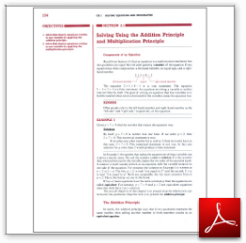Algebra Math Book - Introductory Algebra Thank you for your continual support.

 The power of mathematics rests in the logic of thinking.ID: Sec3-1
Description: Components of an Equation-Addition Principle-Multiplication Principle
Price: 1.99

# Algebra Math book - Introductory Algebra - Chapter 3 - Section 1 - Solving Using the Addition Principle and Multiplication Principle

## Section 3.1 - Objectives

1.  Solve first-degree equations written in one variable by applying the addition principle.

2.  Solve first-degree equations written in one variable by applying the multiplication principle.

Navigate to
Next Section
or Chapter 3 Details or Other Chapters

This section of my algebra math book, Introductory Algebra, also includes in the download:

• Cover Sheet